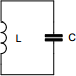# LC-resonance calculator

## Calculates resonance of inductor-capacitor circuit

 Example 1: Must calculate the resonance frequency of a 100nF capacitor and 50uH inductor View example Example 2: Must calculate the inductor to have a resonance of 1Mhz with a 10nF capacitor View example
 Inductance (L) henrys Capacitance (C) farads Frequency hertzYou may use one of the following SI prefix after a value: p=pico, n=nano, u=micro, m=milli, k=kilo, M=mega, G=giga

 Results Inductance ~2.53303 microhenrys